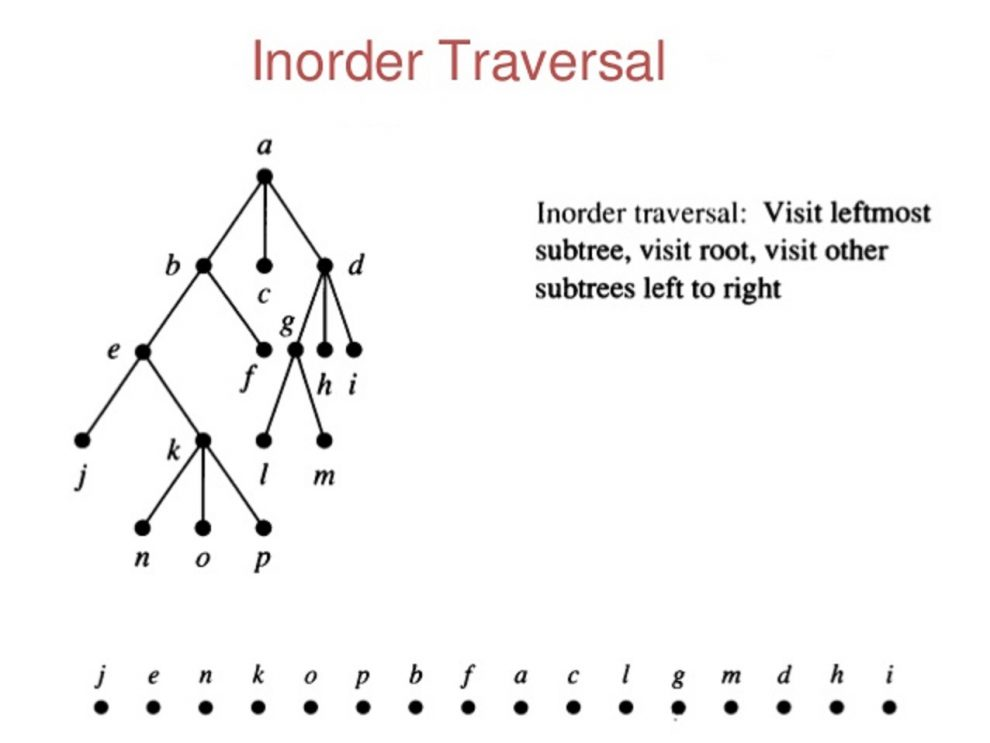Coding

# Java Program – Inorder Tree Traversal without Recursioninorder tree travesal without recursion - Using Stack is the obvious way to traverse tree without recursion.Below is an algorithm for traversing binary tree

Using Stack is the obvious way to traverse tree without recursion. Below is an algorithm for traversing binary tree using stack. See this for step wise step execution of the algorithm.

```1) Create an empty stack S.
2) Initialize current node as root
3) Push the current node to S and set current = current->left until current is NULL
4) If current is NULL and stack is not empty then
a) Pop the top item from stack.
b) Print the popped item, set current = popped_item->right
c) Go to step 3.
5) If current is NULL and stack is empty then we are done.
```

Let us consider the below tree for example

```            1
/   \
2      3
/  \
4     5

Step 1 Creates an empty stack: S = NULL

Step 2 sets current as address of root: current -> 1

Step 3 Pushes the current node and set current = current->left until current is NULL
current -> 1
push 1: Stack S -> 1
current -> 2
push 2: Stack S -> 2, 1
current -> 4
push 4: Stack S -> 4, 2, 1
current = NULL

Step 4 pops from S
a) Pop 4: Stack S -> 2, 1
b) print "4"
c) current = NULL /*right of 4 */ and go to step 3
Since current is NULL step 3 doesn't do anything.

Step 4 pops again.
a) Pop 2: Stack S -> 1
b) print "2"
c) current -> 5/*right of 2 */ and go to step 3

Step 3 pushes 5 to stack and makes current NULL
Stack S -> 5, 1
current = NULL

Step 4 pops from S
a) Pop 5: Stack S -> 1
b) print "5"
c) current = NULL /*right of 5 */ and go to step 3
Since current is NULL step 3 doesn't do anything

Step 4 pops again.
a) Pop 1: Stack S -> NULL
b) print "1"
c) current -> 3 /*right of 5 */

Step 3 pushes 3 to stack and makes current NULL
Stack S -> 3
current = NULL

Step 4 pops from S
a) Pop 3: Stack S -> NULL
b) print "3"
c) current = NULL /*right of 3 */```
``````// non-recursive java program for inorder traversal

/* importing the necessary class */
import java.util.Stack;

/* Class containing left and right child of current
node and key value*/
class Node {

int data;
Node left, right;

public Node(int item) {
data = item;
left = right = null;
}
}

/* Class to print the inorder traversal */
class BinaryTree {

Node root;

void inorder() {
if (root == null) {
return;
}

//keep the nodes in the path that are waiting to be visited
Stack<Node> stack = new Stack<Node>();
Node node = root;

//first node to be visited will be the left one
while (node != null) {
stack.push(node);
node = node.left;
}

// traverse the tree
while (stack.size() > 0) {

// visit the top node
node = stack.pop();
System.out.print(node.data + " ");
if (node.right != null) {
node = node.right;

// the next node to be visited is the leftmost
while (node != null) {
stack.push(node);
node = node.left;
}
}
}
}

public static void main(String args[]) {

/* creating a binary tree and entering
the nodes */
BinaryTree tree = new BinaryTree();
tree.root = new Node(1);
tree.root.left = new Node(2);
tree.root.right = new Node(3);
tree.root.left.left = new Node(4);
tree.root.left.right = new Node(5);
tree.inorder();
}
}``````

Time Complexity: O(n)

READ  Python Programming - Longest Common Subsequence

Output:

` 4 2 5 1 3`#### Venkatesan Prabu

Wikitechy Founder, Author, International Speaker, and Job Consultant. My role as the CEO of Wikitechy, I help businesses build their next generation digital platforms and help with their product innovation and growth strategy. I'm a frequent speaker at tech conferences and events.

X